# Python模拟百度贴吧APP短信登录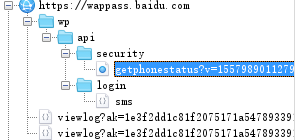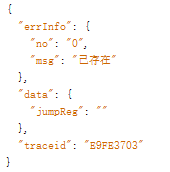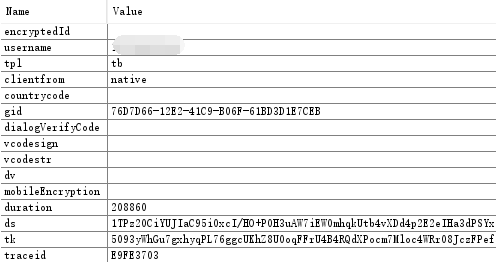### OK，第一步发送验证码步骤分析完了，就来分析以下个个参数。

ds跟tk，直接get上面提到的链接即可得到。

gid的生成方法网上很多，就不分析了，下面是Python代码：

``````def getGID():
def transform(char):
if char == "4" or char == "-": return char
number = random.randint(0, 15)
if char != "x": number = 3 & number | 8
return format(number, "x").upper()
return "".join([transform(c) for c in "xxxxxxx-xxxx-4xxx-yxxx-xxxxxxxxxxxx"])``````

traceid

``````def getTraceid():
traceid = "CA9C8801"
seed = "1234567890abcdefghijklmnopqrstuvwxyz"
sa = []
for i in range(8):
sa.append(random.choice(seed))
traceid = ''.join(sa).upper()
return traceid``````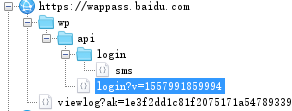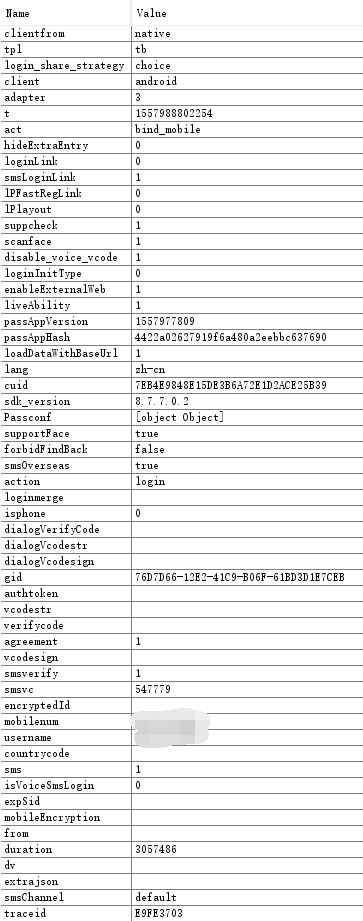``````{
"errInfo": {
"no": "0",
}***省略``````

``````vcodesign = r['data']['vcodesign']
vcodestr = r['data']['vcodestr']
print('需要验证码')
vcodeurl = f'https://wappass.baidu.com/cgi-bin/genimage?{vcodestr}'``````

## 1 条评论

•非技术的路过。